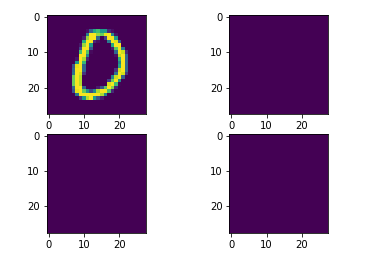# Activation values in ResNet

Hello again! now for a neuron x in the layer `fc2` I’d like to plot the activation value tensor. I want to see all the values for the batch. How do I do this?

You could reshape the activation stored in the forward hook and visualize it e.g. via `matplotlib.pyplot.plot`.

I don’t know how to do it. can you’re please elaborate?

You can use the linked code from my previous reply and use `matplotlib` directly:

``````class MyModel(nn.Module):
def __init__(self):
super(MyModel, self).__init__()
self.cl1 = nn.Linear(25, 60)
self.cl2 = nn.Linear(60, 16)
self.fc1 = nn.Linear(16, 120)
self.fc2 = nn.Linear(120, 84)
self.fc3 = nn.Linear(84, 10)

def forward(self, x):
x = F.relu(self.cl1(x))
x = F.relu(self.cl2(x))
x = F.relu(self.fc1(x))
x = F.relu(self.fc2(x))
x = F.log_softmax(self.fc3(x), dim=1)
return x

activation = {}
def get_activation(name):
def hook(model, input, output):
activation[name] = output.detach()
return hook

model = MyModel()
model.fc2.register_forward_hook(get_activation('fc2'))
x = torch.randn(1, 25)
output = model(x)
print(activation['fc2'])

plt.imshow(activation['fc2'])
``````

It gives me this error:

Invalid shape (1000, 32, 32) for image data.

this tensor is the activation of a neuron x in layer `fc2`.

You cannot plot this tensor via `imshow` as described in your other topic.

how can I make 1000 plots of 32*32?

This should work, but given that you are trying to plot a lot of subplots, you might want to increase the figure size etc.:

``````x = torch.randn(1000, 32, 32)
fig, axs = plt.subplots(20, 50)
for ax, x_ in zip(axs.flatten(), x):
ax.imshow(x_)
``````

I am getting this outputon editing sub plot to 2* 2 only getting first imageAs mentioned in the previous post:

E.g. check the `figsize` argument.

1 Like

Hi Ptrblck,

I have used this solution. My batch size is 4, and I extracted the intermediate layer of my model.

I am getting the resultant output with shape(4,84). However, I am wondering 4 means that I only extract 1 batch activation value. How can I extract all batch activation values? Can you please help me out in this regard?

If you want to get the activations for all samples, you could append them to e.g. an `activation` `list` instead of replacing them in the `dict`.

activation = {}
def get_activation(name):
def hook(model, input, output):
activation[name] = output.detach().numpy()
return hook

save_net.fc2.register_forward_hook(get_activation(‘fc2’))
for batch_idx, (x, y) in enumerate(trainloader):
save_net(x)

I replaced it with this

activations = []
def get_activation(name):
def hook(model, input, output):
activation= output.detach().numpy()
activations.append(activation)

``````return hook
``````

Is it correct?

Yes, this looks right (although the code is not properly formatted, but I assume it’s correct in your script). Did you try it out and was it working?

Yep, I tried out.

I was trying to fetch out layer three activation values:
Linear(in_features=84, out_features=10, bias=True)

I am now getting this shape of data (images, 4, 10). Is it correct to reshape the array like this:

(images, 4*10) ?

Linear(in_features=84, out_features=10, bias=True)

It seems you are passing the inputs to the linear layer as `[batch_size, 4, 84]` which could be interpreted as temporal data where the linear layer is applied to each time step (dim1).
Does this fit your use case as usually you would flatten the activation before passing it to the linear layer via:

``````x = x.view(x.size(0), -1)
out = linear(x)
``````

I have already flattened the input before passing it to the linear layer.

flatten_values = torch.flatten(con_2_output, 1)
after flattening, these are the three linear layers, and I want to extract the last layer’s feature activation values. Do I need to flatten again before fetching the values from 2 or third linear layer?

(fc1): Linear(in_features=400, out_features=120, bias=True)
(fc2): Linear(in_features=120, out_features=84, bias=True)
(fc3): Linear(in_features=84, out_features=10, bias=True)

Something seems to be off then, since the flattened activation should have two dimensions instead of `[images, 4, 10]`. Add a `print` statement to the `forward` method of your model and check why the activation is 3-dimensional.

No, you do not need to flatten the activations again between the linear layers, as the outputs should already have 2 dimensions.

After extracting the activations, I am converting them into a NumPy array. So before converting into the Numpy, the shape is, let’s say (80,10), and after converting into NumPy, it is (1, 80, 10). Why is it so?

That should also not happen as seen in e.g. these code snippets:

``````x = torch.randn(1, 2, 3)
arr = x.numpy()
print(arr.shape)
# (1, 2, 3)

x = torch.randn(80, 10)
arr = x.numpy()
print(arr.shape)
# (80, 10)

x = torch.randn(1, 80, 10)
arr = x.numpy()
print(arr.shape)
# (1, 80, 10)
``````

PyTorch will not change the shape of this tensor behind your back.